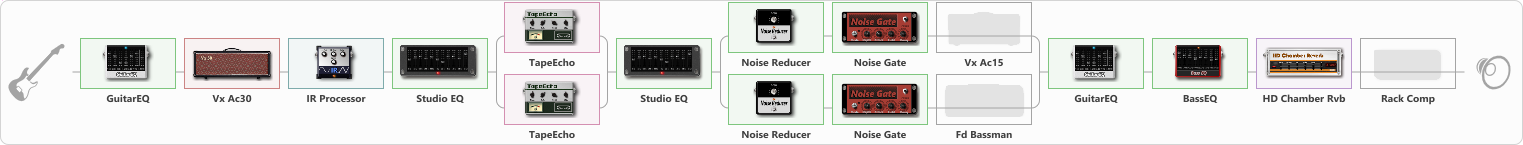# clean noel Hendriks

Discussion in 'ToneLib-GFX presets' started by Noel Hendriks, Sep 6, 2021.

1. clean noel Hendriks

Preset name: Last Date - The Spotnicks

wwwwwwwwwwwwwwooooooooooooooooooooooooooooowwwwwwwwwwwwwwwwwwww

Effects chain:Effect: "GuitarEQ" (Dynamics / Filter), active - "yes"
{
"160 Hz" = 0
"400 Hz" = 0
"800 Hz" = 0
"1.6 kHz" = 0
"3.2 kHz" = 0
"6.4 kHz" = 0
"12 kHz" = 0
"Level (dB)" = 0
}

Effect: "Vx Ac30" (Amp simulators), active - "yes"
{
"Gain" = 20
"Bass" = 100
"Middle" = 0
"Treble" = 0
"Presence" = 100
"Master" = 100
"Level (dB)" = 10
}

Effect: "IR Processor" (Cabinets), active - "yes"
{
"IR" = Cab IR Sample4
"Low Cut (Hz)" = 0
"Hi Cut (kHz)" = 20.0
"Mix" = 100
"Level (dB)" = 11
}

Effect: "Studio EQ" (Dynamics / Filter), active - "yes"
{
"31 Hz" = -9
"62 Hz" = 0
"125 Hz" = -3
"250 Hz" = 2
"500 Hz" = 10
"1 kHz" = -5
"2 kHz" = -1
"4 kHz" = 11
"8 kHz" = 10
"16 kHz" = -6
"Level (dB)" = -3
}

Effect: "Splitter" (Dynamics / Filter)
{
"A-Bypass" = Off
"A-Pan" = 0
"A-Level" = 55
"B-Bypass" = Off
"B-Pan" = 0
"B-Level" = 55

'A' branch:
{

Effect: "TapeEcho" (Delay), active - "yes"
{
"Time" = 100
"Feedback" = 0
"Tone" = 54
"LoDamp" = 35
"Mix" = 57
}
}
'B' branch:
{

Effect: "TapeEcho" (Delay), active - "yes"
{
"Time" = 200
"Feedback" = 66
"Tone" = 65
"LoDamp" = 31
"Mix" = 81
}
}
}

Effect: "Studio EQ" (Dynamics / Filter), active - "yes"
{
"31 Hz" = -15
"62 Hz" = -8
"125 Hz" = 4
"250 Hz" = 2
"500 Hz" = -10
"1 kHz" = -1
"2 kHz" = 8
"4 kHz" = 9
"8 kHz" = -12
"16 kHz" = -12
"Level (dB)" = 0
}

Effect: "Splitter" (Dynamics / Filter)
{
"A-Bypass" = Off
"A-Pan" = 0
"A-Level" = 55
"B-Bypass" = Off
"B-Pan" = 0
"B-Level" = 55

'A' branch:
{

Effect: "Noise Reducer" (Dynamics / Filter), active - "yes"
{
"Sens" = 78
"Mode" = Soft
}

Effect: "Noise Gate" (Dynamics / Filter), active - "yes"
{
"Mode" = Auto
"Depth" = 50
"Threshold" = 50
"Attack" = 98
"Hold" = 683
"Decay" = 354
}

Effect: "Vx Ac15" (Amp simulators), active - "no"
{
"Gain" = 20
"Bass" = 100
"Middle" = 100
"Treble" = 16
"Presence" = 100
"Master" = 100
"Level (dB)" = 0
}
}
'B' branch:
{

Effect: "Noise Reducer" (Dynamics / Filter), active - "yes"
{
"Sens" = 69
"Mode" = Soft
}

Effect: "Noise Gate" (Dynamics / Filter), active - "yes"
{
"Mode" = Auto
"Depth" = 50
"Threshold" = 50
"Attack" = 100
"Hold" = 556
"Decay" = 585
}

Effect: "Fd Bassman" (Amp simulators), active - "no"
{
"Gain" = 13
"Bass" = 100
"Middle" = 100
"Treble" = 6
"Presence" = 100
"Master" = 100
"Level (dB)" = 0
}
}
}

Effect: "GuitarEQ" (Dynamics / Filter), active - "yes"
{
"160 Hz" = -7
"400 Hz" = 3
"800 Hz" = 1
"1.6 kHz" = -3
"3.2 kHz" = -6
"6.4 kHz" = -3
"12 kHz" = 0
"Level (dB)" = 0
}

Effect: "BassEQ" (Dynamics / Filter), active - "yes"
{
"50 Hz" = 8
"120 Hz" = 3
"400 Hz" = -6
"500 Hz" = -8
"800 Hz" = 3
"4.5 kHz" = 5
"10 kHz" = 6
"Level (dB)" = 1
}

Effect: "HD Chamber Rvb" (Reverberation), active - "yes"
{
"Time" = 6.4
"PreLPF" = 100
"PreDelay" = 284
"HiDamp" = 9
"LoGain" = 12.0
"Mix" = 23
}

Effect: "Rack Comp" (Dynamics / Filter), active - "no"
{
"Threshold (dB)" = -18
"Ratio" = 7
"Attack" = Fast
"Release (ms)" = 386
"Knee" = 50
"Level (dB)" = 10
}

Note: You will need to download and install the ToneLib-GFX software to use the preset.

#### Attached Files:

• ###### clean_noel_Hendriks.tlgfx
File size:
5.1 KB
Views:
2,386
Jack Disney likes this.